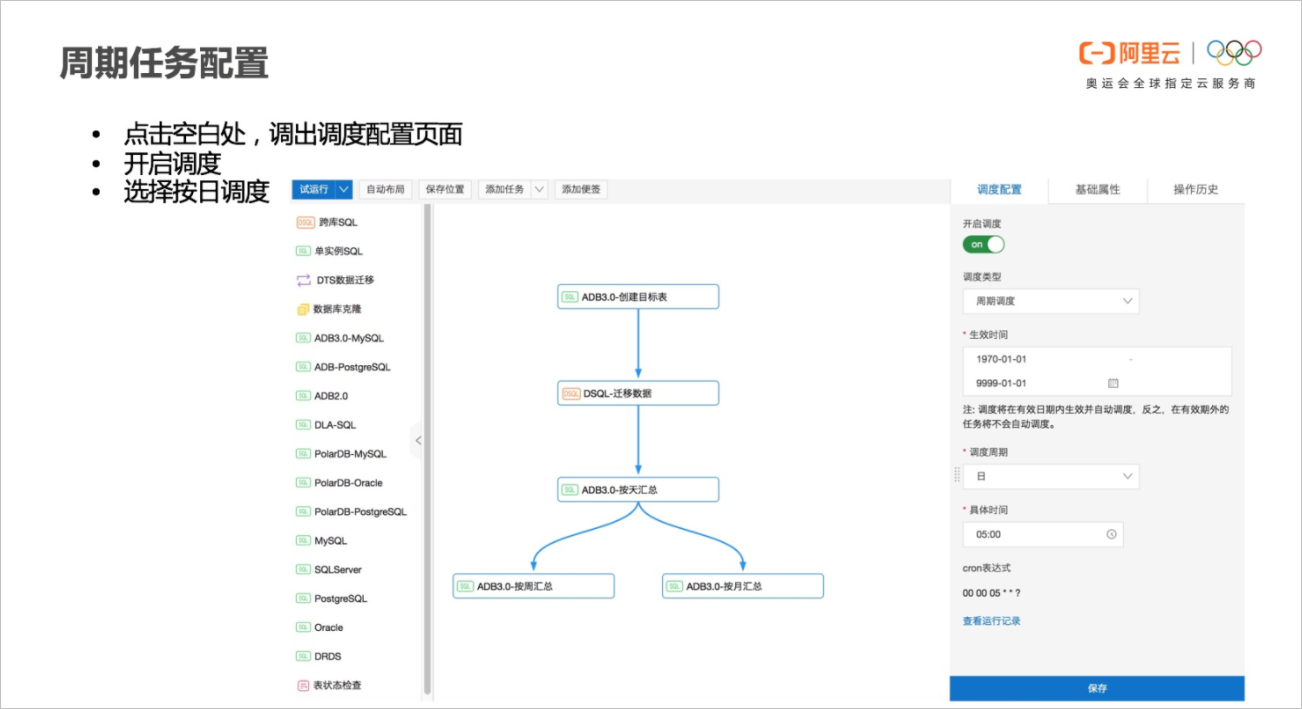## 背景信息

DMS是阿里云提供的数据操作、数据安全管理以及数据开发的Web服务平台。DMS提供数据库客户端的功能，支持多种数据源（MySQL，PostgreSQL，SQLServer，Oracle，Redis以及MongoDB等），实现统一的权限管理，支持数据库稳定的变更，同时集成了数据开发功能（包括数仓开发模式和任务编排模式）。## DMS数据开发使用场景

DMS的数据开发能够用于多种场景，包括：
• 离线数据：T+1报表。
• 实时数据：五分钟实时报表。
• 智能数据：直接对接AI计算等框架。
• 冷数据：冷数据OSS定期备份。
• 事务数据：大批量数据定期删除、更新。

## 案例背景

``````CREATE TABLE rds_table(
id INT,
price DECIMAL(10,2),
trx_time TIMESTAMP
);``````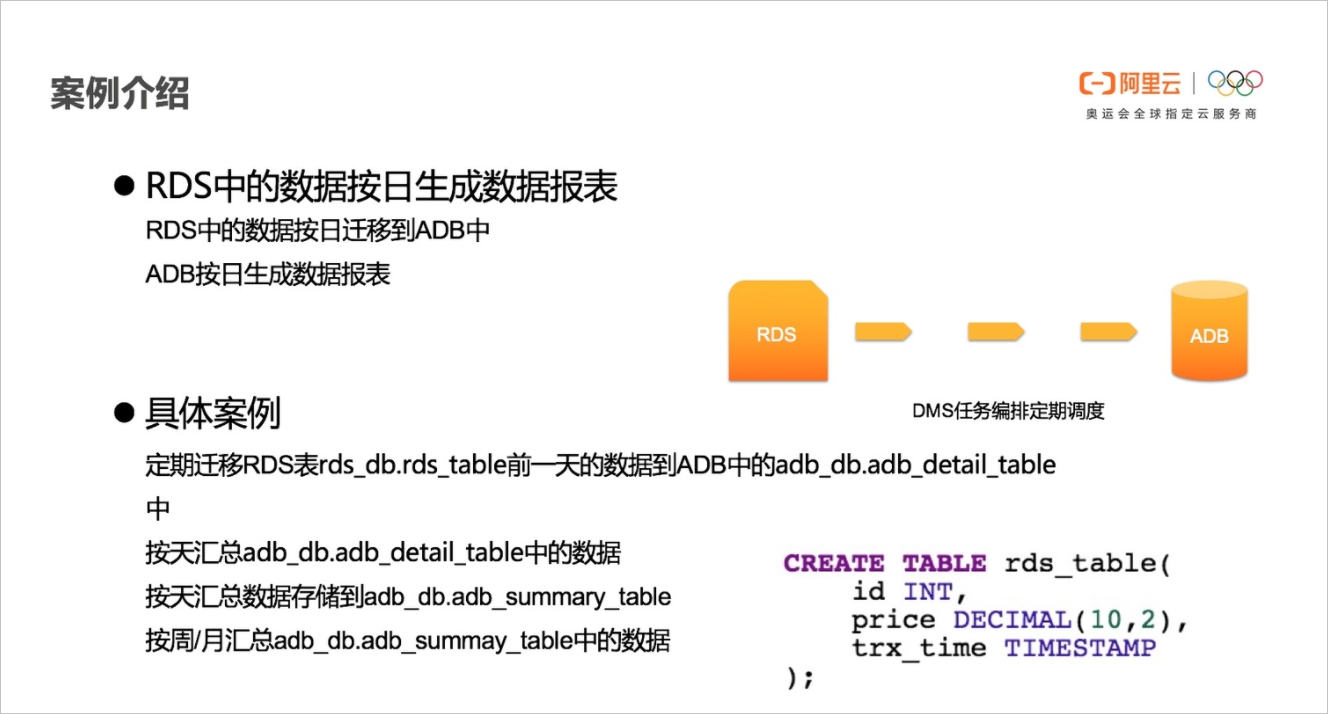## 准备工作

``````insert into rds_table values(1, 10.9, date_add(now(), interval -1 day));
insert into rds_table values(2, 20.9, date_add(now(), interval -1 day));
insert into rds_table values(3, 30.9, date_add(now(), interval -1 day));
insert into rds_table values(4, 40.9, date_add(now(), interval -1 day));
insert into rds_table values(5, 50.9, now());
insert into rds_table values(6, 60.9, now());
insert into rds_table values(7, 70.9, now());
insert into rds_table values(8, 80.9, now());``````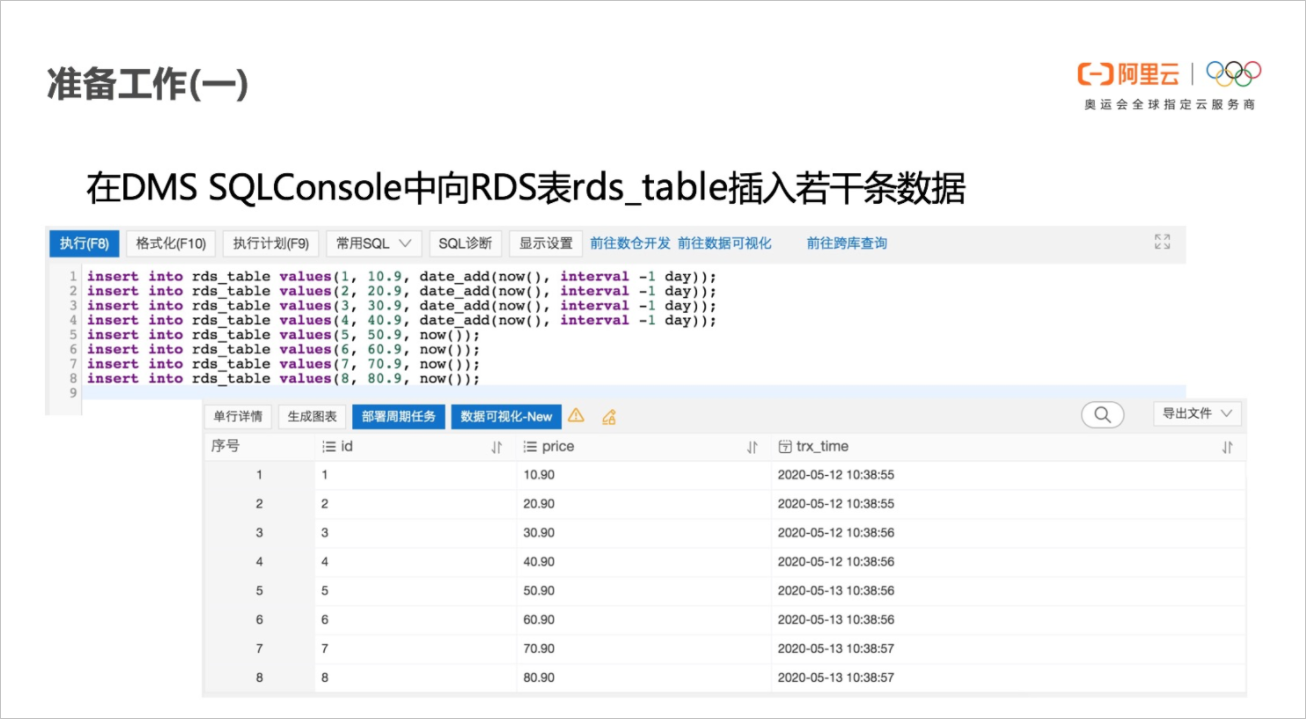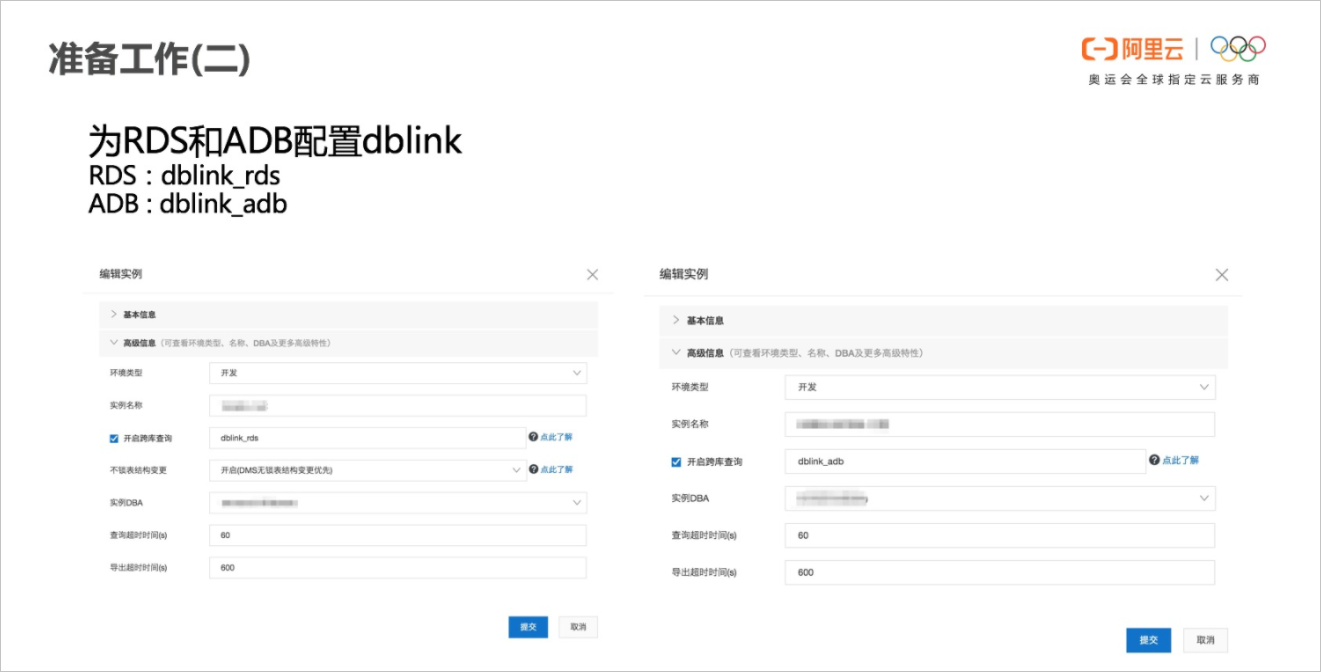## 实现任务流

1. 建立新任务流。
在DMS中的数据工厂/任务编排里，建立一个新的任务流：daily_weekly_monthly_report。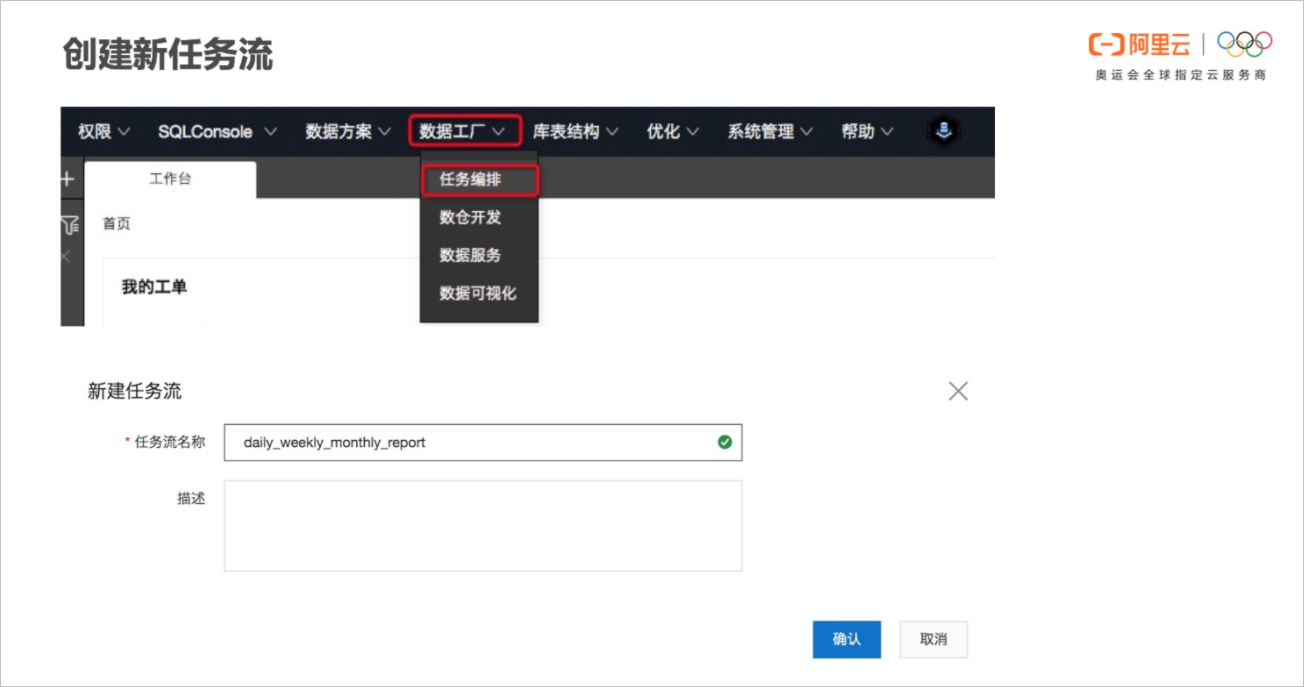2. 创建任务节点。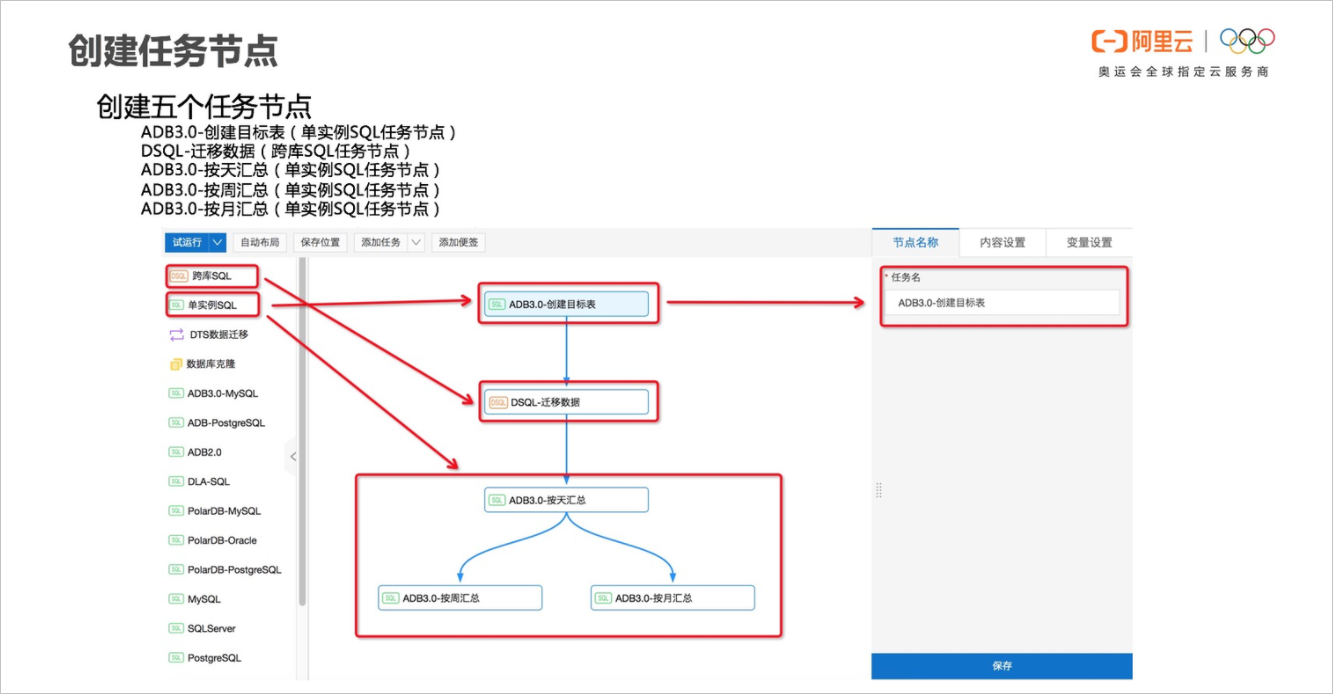``````CREATE TABLE IF NOT EXISTS `adb_detail_table` (
`dt` DATE, `id` INT, `price` DECIMAL(10,2), `trx_time` datetime
);

CREATE TABLE IF NOT EXISTS `adb_summary_table` (
`dt` DATE, `total` DECIMAL(1000,2)
);

CREATE TABLE IF NOT EXISTS `adb_weekly_table` (
`weekstart` DATE, `weekend` DATE, `total` DECIMAL(1000,2)
);

CREATE TABLE IF NOT EXISTS `adb_monthly_table` (
`monthdate` DATE, `total` DECIMAL(1000,2)
);``````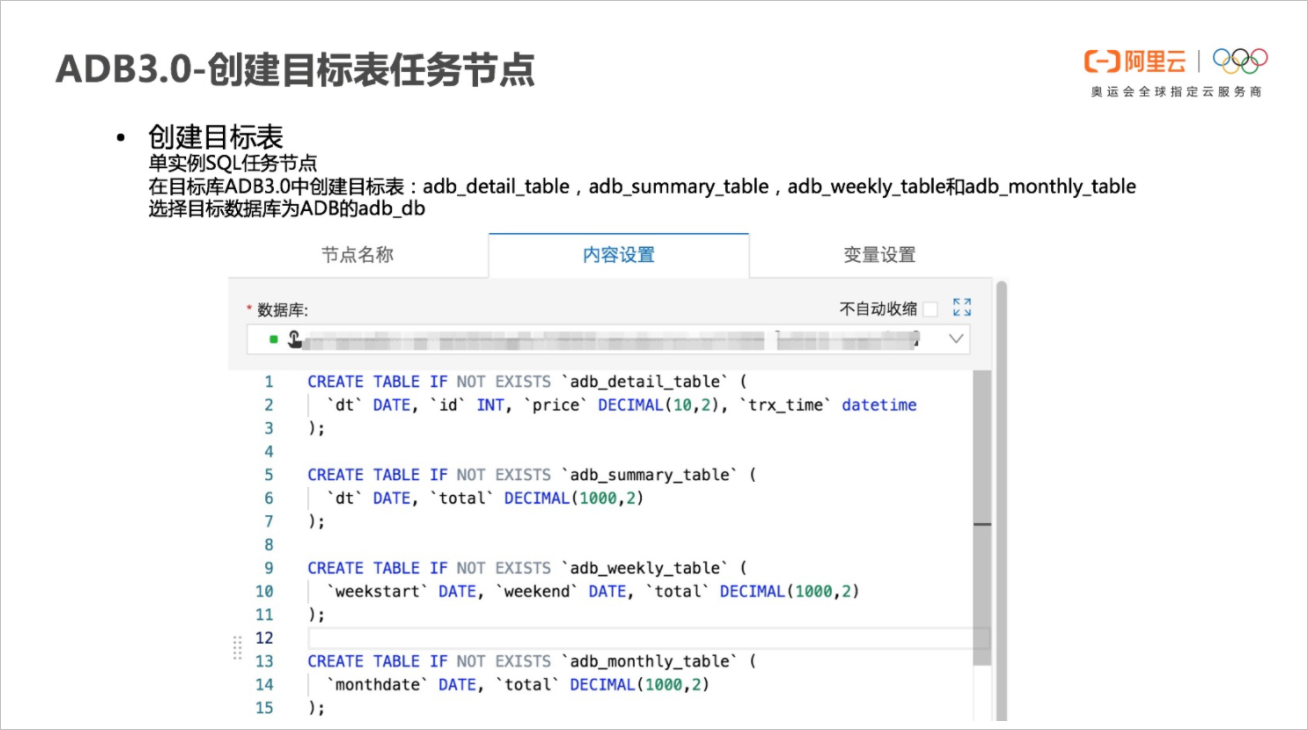4. 创建DSQL-迁移数据任务节点。
DSQL-迁移数据任务节点是一个跨库SQL任务节点，实现该任务节点需要先配置时间变量，然后使用跨库SQL实现数据迁移。
• 配置时间变量。

需要两个时间变量，它们分别是：

• bizdate：当前日期前一天的日期（为系统默认变量）。
• today：当前日期。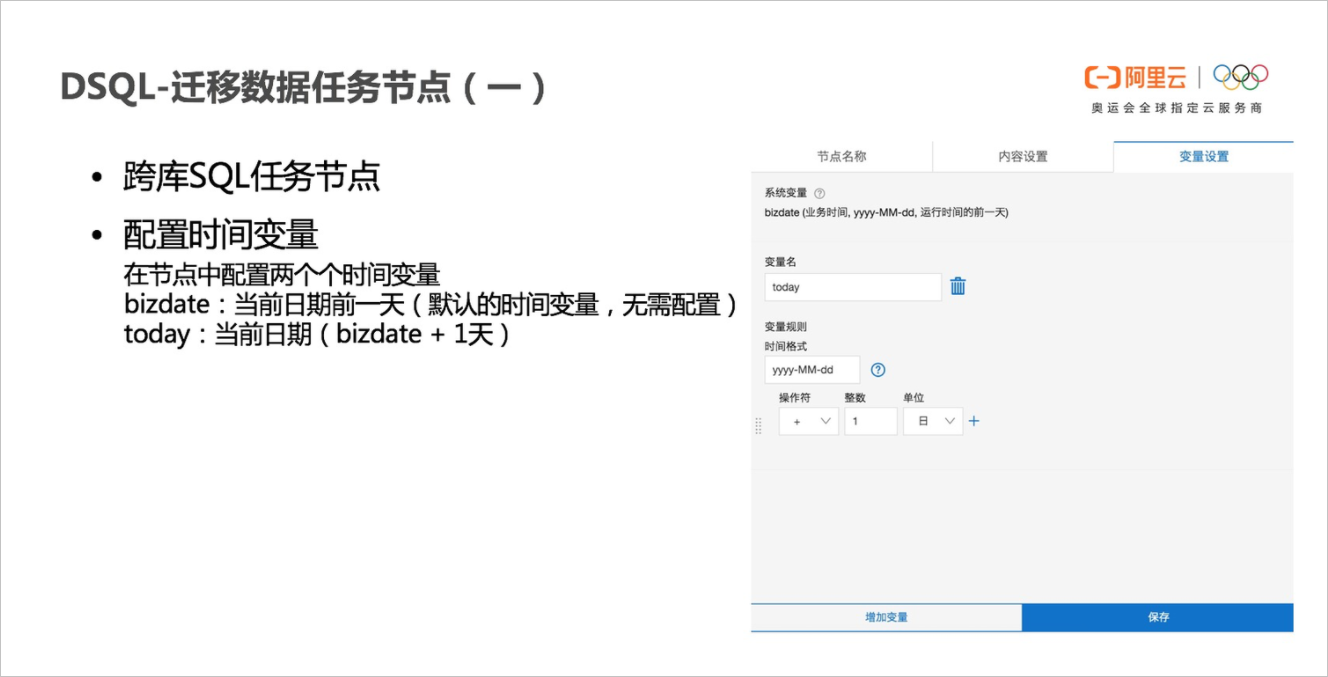• 数据迁移SQL。

``````INSERT INTO `dblink_adb`.`adb_db`.`adb_detail_table` (`dt`, `id`, `price`, `trx_time`)
SELECT '\${bizdate}', `id`, `price`, `trx_time`
WHERE `trx_time` >= '\${bizdate}'
AND `trx_time` < '\${today}';``````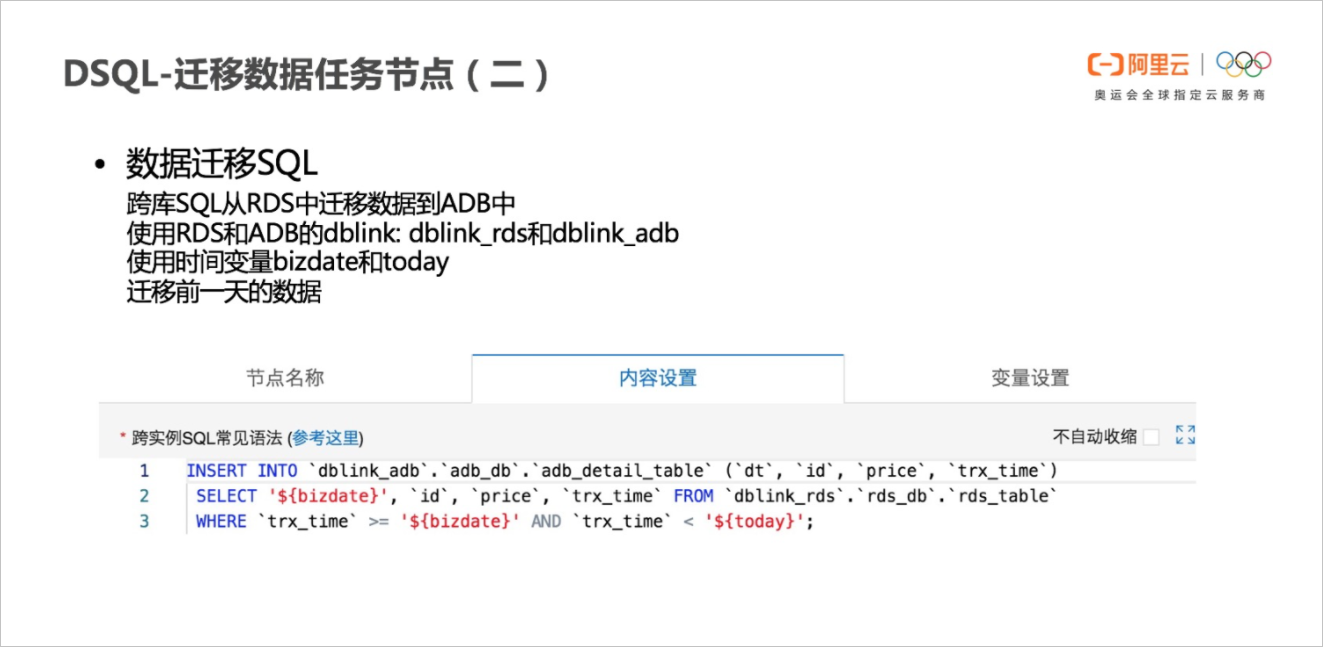``````INSERT INTO `adb_summary_table` (`dt`, `total`)
SELECT `dt`, sum(`price`) AS total
WHERE `dt` = '\${bizdate}'
GROUP BY `dt`;``````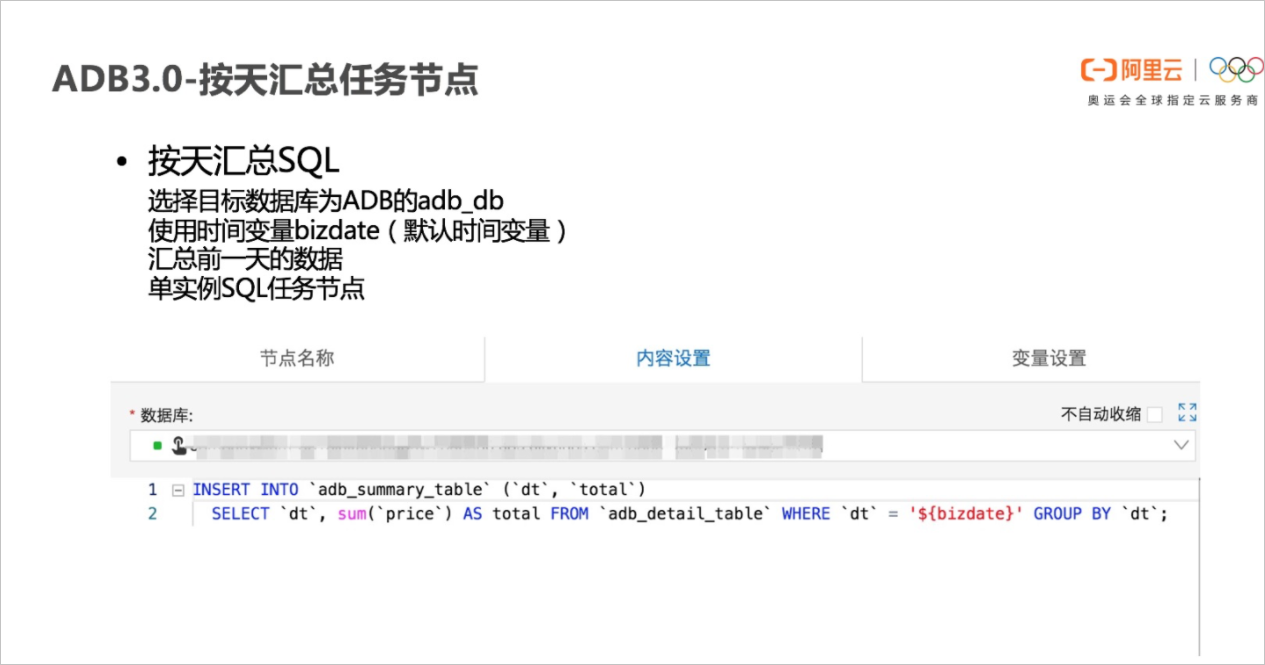• 配置时间变量。
需要三个时间变量，它们分别是：
• bizdate：当前日期前一天的日期（为系统默认变量）。
• today：当前日期。
• weekstart: 当前日期前七天（bizdate~6天）。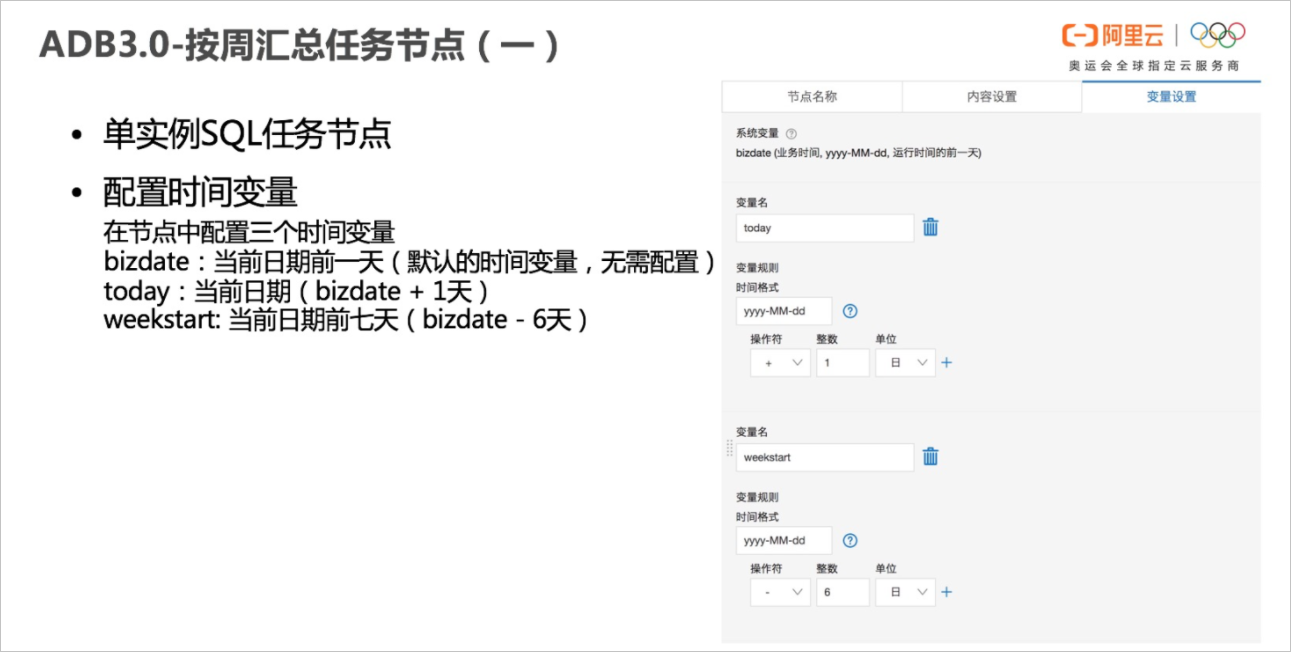• 按周汇总SQL。

``````INSERT INTO `adb_weekly_table` (`weekstart`, `weekend`, `total`)
SELECT *
FROM(
SELECT '\${weekstart}', '\${bizdate}', sum(`total`) AS total
WHERE `dt` < '\${today}' and
`dt` >= '\${weekstart}' and
date_format('\${today}','%w') = 1) t
WHERE t.total IS NOT null;``````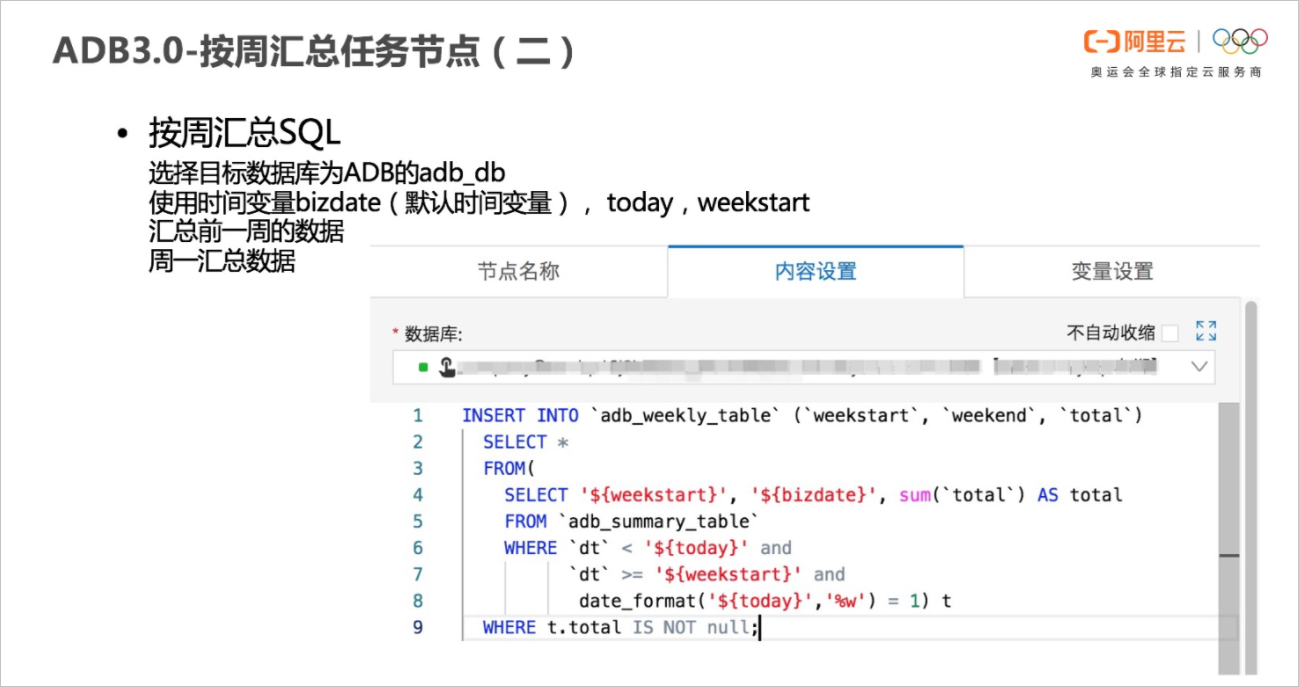• 配置时间变量。
需要三个时间变量，它们分别是：
• bizdate：当前日期前一天的日期（为系统默认变量）。
• today：当前日期。
• monthdate：当前日期前一天所在的年月。
• monthstart: 当前日期前一天所在月第一天的日期。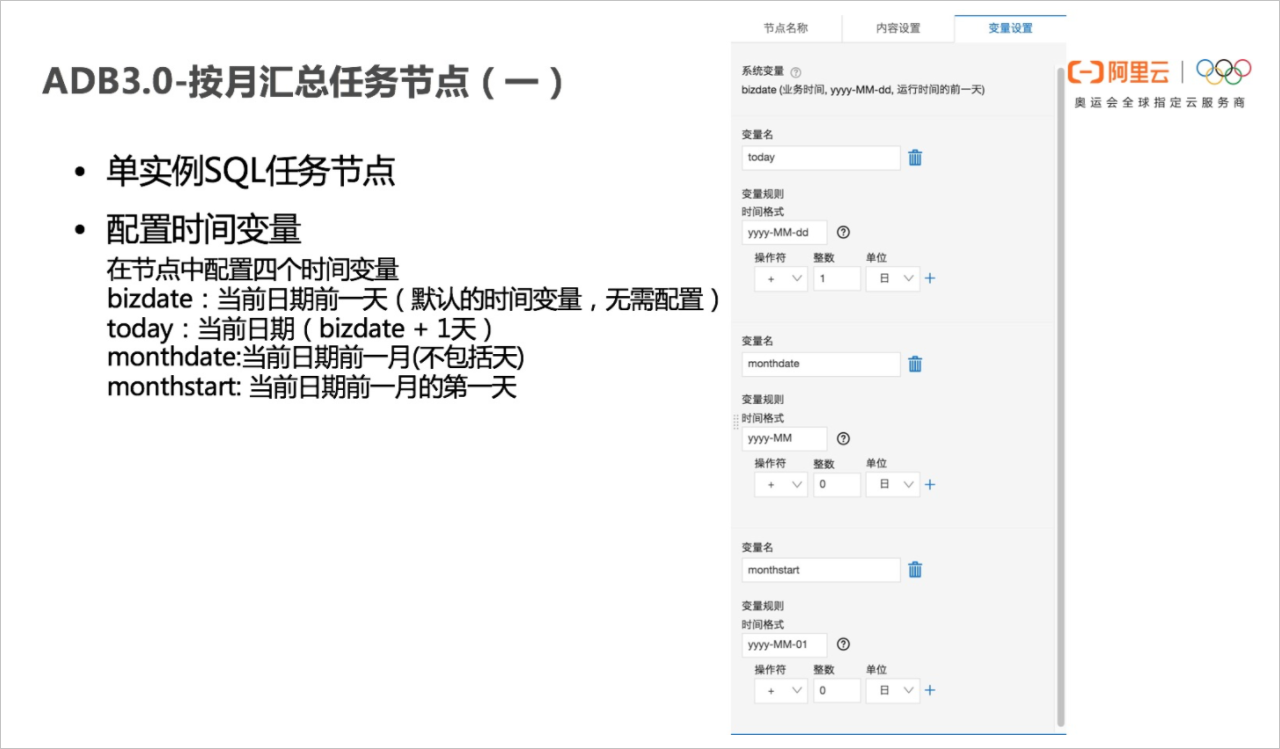• 按月汇总SQL。

``````INSERT INTO `adb_monthly_table` (`monthdate`, `total`)
SELECT *
FROM(
SELECT '\${monthdate}', sum(`total`) AS total
WHERE `dt` < '\${today}' and
`dt` >= '\${monthstart}' and
date_format('\${today}','%d') = 1) t
WHERE t.total IS NOT null;``````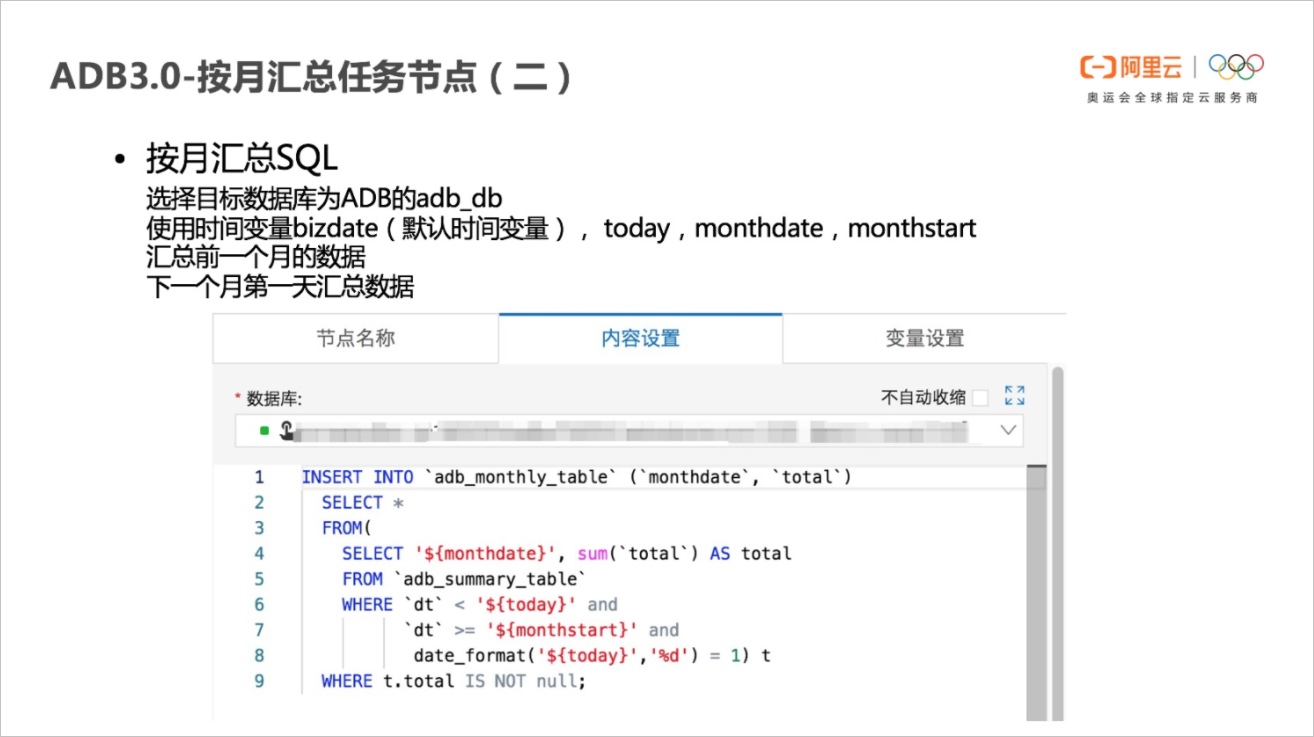8. 运行任务流。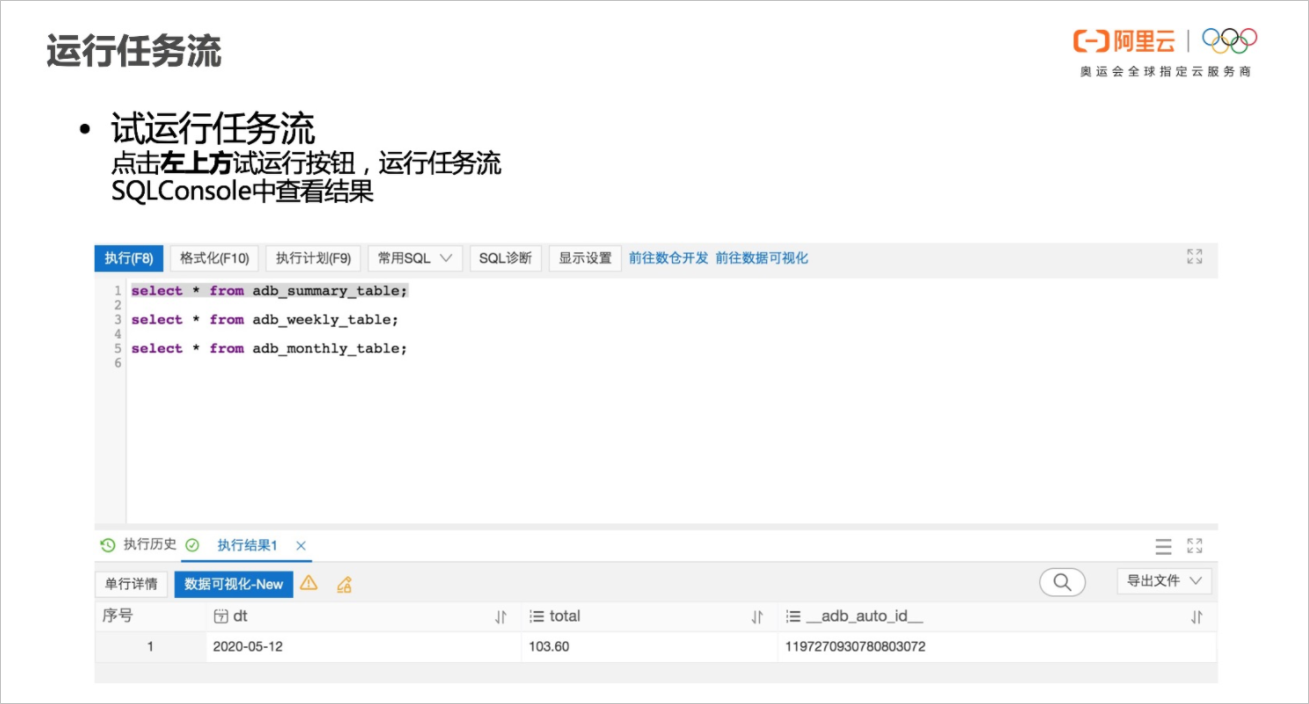9. 周期调度配置。

试运行确定任务流正确以后，点击任务流空白处，调出调度配置页面，设置每天凌晨5点定期调度运行该任务流。